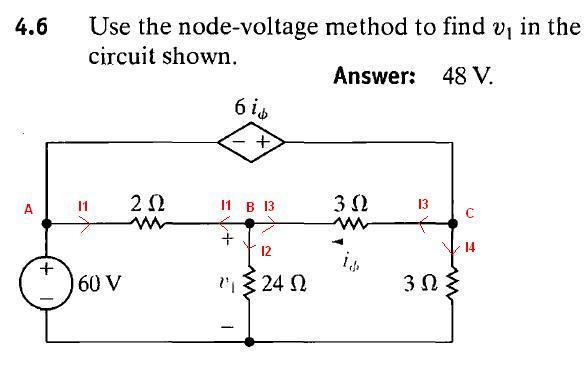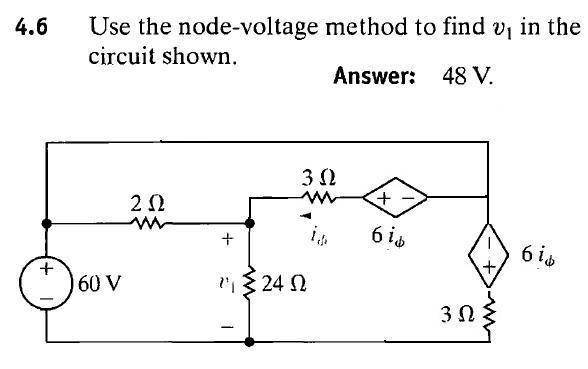# Sloving a depentant voltage source problem using node voltage

Hi everyone,

I currently stumped in the process of approaching a dependent voltage source problem using node voltages. Attached is the circuit.

What I know:

the Node north of the 60V supply with also be 60V

there are four nodes, two when supernodes are used

with the super node being the 6iψ, KCL states the sums must be zero

when I try to solve, I end up getting the equation (using v2 as the junction of the dependent, and both 3Ω resistors):

[(60-v1)/2]+[(V2-V3)/3]+[V2/3]=0
I am not sure how to calculate the current across the 60Volt sourceIn addition, I end up having a recursion problem trying to solve for iψ

Since V2 is 60+6iψ, and iψ is ([V2-V2]/3), I end up with

V2=60+6([V2-V3]/3)

any help would be greatly appreciated

Joe

#### Attachments

•3.9.jpg
28.2 KB · Views: 396

Simply treat A and C as a one big (super) node.
So we only have one unknown node VbCurrent leaving the node is equal current entering the node.

For node B

Vb/24 + (Vb - 60)/2 + (Vb - Vc)/3 = 0 (1)

Vc = Va + 6*Iψ = Va + 6*(Vc - Vb)/3 = 2Vb-Va = 2Vb - 60 (2)

So we have

Vb/24 + (Vb - 60)/2 + (Vb - (2Vb - 60))/3 = 0

http://www.wolframalpha.com/input/?i=+b/24+++(b+-+60)/2+++(b+-+(2b+-+60))/3+=+0

But we can remove the supernode from the circuit. We you can transform this circuit, legally, and get a new circuit which is equivalent to the old oneLast edited: